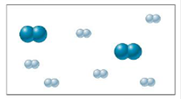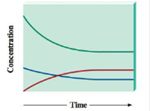# Consider an initial mixture of N 2 and H 2 gases that can be represented as follows: The gases react to form ammonia gas (NH 3 ) as represented by the following concentration profile: a . Label each plot on the graph as N 2 , H 2 , or NH 3 , and explain your answers. b . Explain the relative shapes of the plots. c . When is equilibrium reached? How do you know?### Chemistry: An Atoms First Approach

2nd Edition
Steven S. Zumdahl + 1 other
Publisher: Cengage Learning
ISBN: 9781305079243

#### Solutions

Chapter
Section### Chemistry: An Atoms First Approach

2nd Edition
Steven S. Zumdahl + 1 other
Publisher: Cengage Learning
ISBN: 9781305079243
Chapter 12, Problem 10Q
Textbook Problem
12 views

## Consider an initial mixture of N2 and H2 gases that can be represented as follows:The gases react to form ammonia gas (NH3) as represented by the following concentration profile:a. Label each plot on the graph as N2, H2, or NH3, and explain your answers.b. Explain the relative shapes of the plots.c. When is equilibrium reached? How do you know?

Interpretation Introduction

(a)

Interpretation: A representation depicting the initial mixture of N2 and H2 gases and a concentration profile representing the reaction of the stated gases to form ammonia is given. The questions based on the given data are to be answered.

Concept introduction: The state when the reactants involved in a chemical reaction and the products formed in the reaction exist in concentrations having no further tendency to change is known as an equilibrium state of the reaction.

To determine: The labeling of the given terms and an explanation regarding the same.

### Explanation of Solution

Given

The initial moles of N2 present are 2 .

The initial moles of H2 present are 6 .

A balanced chemical equation for the given reaction is,

2N2

Interpretation Introduction

(b)

Interpretation: A representation depicting the initial mixture of N2 and H2 gases and a concentration profile representing the reaction of the stated gases to form ammonia is given. The questions based on the given data are to be answered.

Concept introduction: The state when the reactants involved in a chemical reaction and the products formed in the reaction exist in concentrations having no further tendency to change is known as an equilibrium state of the reaction.

To determine: An explanation regarding the relative shapes of the given plot.

Interpretation Introduction

(c)

Interpretation: A representation depicting the initial mixture of N2 and H2 gases and a concentration profile representing the reaction of the stated gases to form ammonia is given. The questions based on the given data are to be answered.

Concept introduction: The state when the reactants involved in a chemical reaction and the products formed in the reaction exist in concentrations having no further tendency to change is known as an equilibrium state of the reaction.

To determine: The point when the equilibrium state is reached.

### Still sussing out bartleby?

Check out a sample textbook solution.

See a sample solution

#### The Solution to Your Study Problems

Bartleby provides explanations to thousands of textbook problems written by our experts, many with advanced degrees!

Get Started

Find more solutions based on key concepts
On average, young men in the United States obtain percent of their calories from beverages. a. 12 b. 22 c. 32 d...

Nutrition: Concepts and Controversies - Standalone book (MindTap Course List)

In what way has biotechnology had an impact on agriculture in the United States?

Human Heredity: Principles and Issues (MindTap Course List)

What are the chemical differences between DNA and RNA?

Biology: The Dynamic Science (MindTap Course List)

An electric power plain that would make use of the temperature gradient in the ocean has been proposed. The sys...

Physics for Scientists and Engineers, Technology Update (No access codes included)